# Investigation 4 – Lab### MINDSET

This Investigation is designed to:

• promote your investigation of a fourth way in which light interacts with the medium, refraction,
• provide you an opportunity to explore the behavior of light waves as they enter and exit different mediums including air, water and oil,
• measure angles of incidence and angles of refraction and use Snell’s Law to calculate the index of refraction of various media, and
• help you understand the relationship between the index of refraction and the extent to which light is refracted by a medium.

### BE PREPARED

Student Preparation for the Investigation includes having students gather the following materials. This preparation takes place on lab day after student lab groups have settled at their assigned lab tables.

Note: The materials are listed in students’ SDRs. They are also listed below for your reference.

• (1) 400 ml beaker filled with 400 ml of water
• (1) 400 ml beaker filled with 400 ml of oil
• (1) 400 ml beaker, empty
• (4) straight pins
• (1) 6cm x 12cm piece of cardboard
• (1) acrylic semi-circle
• (1) refraction tank
• (1) protractor
• (1) glass slide
• (1) concave lens
• (1) convex lens
• (1) calculator
• (3) blank sheets of paper
• (1) metric ruler

Direct one student from each lab group to collect the materials listed in their SDRs.

### INVESTIGATE

• You will investigate the refraction of light through three different mediums, determine the index of refraction for each medium, and observe any changes in the way in which objects appear when viewed through the medium. In addition to air, the three media you will investigate are an acrylic solid, water, and oil.
• Reflect on the PreLab video as you move through the procedural steps.
• During the Experiment, every procedural step is important. If one step is skipped, data can become invalid. To help you keep on track, read each step thoroughly, complete the step, then check it off (Read it – Do it – Check it).
• Complete all of the procedural steps in your SDR.

Note: The procedural steps are listed below for your reference.

Trial 1: Refraction through an acrylic solid

1. Place one piece of clean, white paper on the table.
2. Make a tracing of your medium:

A. Place the acrylic semicircle in the center of the paper.

B. Trace the outline of the acrylic semicircle on the piece of paper. Your tracing should look like this: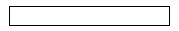1. Use the ruler to draw an 8 cm line approximately 1/3 from the left side of the tracing. The line should be perpendicular to the long side of the tracing. Your diagram should look like this: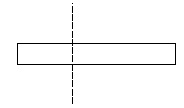1. Simulate a light ray entering the acrylic cylinder at an angle of 30° to the perpendicular line.

A. Use the protractor to measure a 30o angle from the perpendicular line. Place a dot at the 30o mark.

B. Draw a line from the intersection of the perpendicular line through the dot. Your diagram should look like this: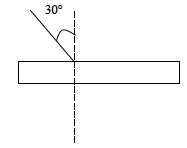C. Place the cardboard directly under your tracing.

D. Take two straight pins and place them along two points of the 30° line.

E. Place the pins approximately 1 cm from each end of the line. Insert them through the paper so they stick into the cardboard.

1. Place the semicircle back into its outline.
2. Lower yourself to eye level with the semicircle, so that the two straight pins are behind one another and they appear as one pin.
3. Line up two more pins on the opposite side of the semicircle, so that all four pins appear to be in the same line. This simulates how a light wave travels from the air, through the semicircle, and back through the air to your eyes.
4. Remove the semicircle from the diagram.
1. What does the line formed by the pins look like?
1. Take out the four pins and remove the cardboard.
2. Use a ruler to line up the two new pinholes.
3. Draw a line that connects the two pins holes and intersects with the outline of the semicircle.
4. Indicate on the diagram the light wave in the air and when it is in the acrylic semicircle. Your complete refraction diagram should appear similar to the diagram below: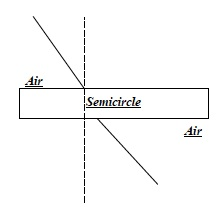1. What do you think will happen when the two lines drawn outside of the medium are connected?
2. Using a different colored pen or pencil, connect the two outer lines within the area inside the acrylic medium.
1. What do you see?
1. In order to calculate the index of refraction, you will need to determine the angle of incidence and the angle of refraction. Use the diagram you created for yourself on the blank piece of white paper.
2. An example of a completed diagram is located below to help you with the procedure.

A. Label the perpendicular line drawn on the medium with a “P.”

B. Label the angle of incidence.

C. Use the protractor to measure the angle of incidence.

D. Use the different color pen to extend the line inside the medium out of the medium so that you can better determine the angle of refraction.

E. Label the angle of refraction.

F. Use the protractor to measure the angle.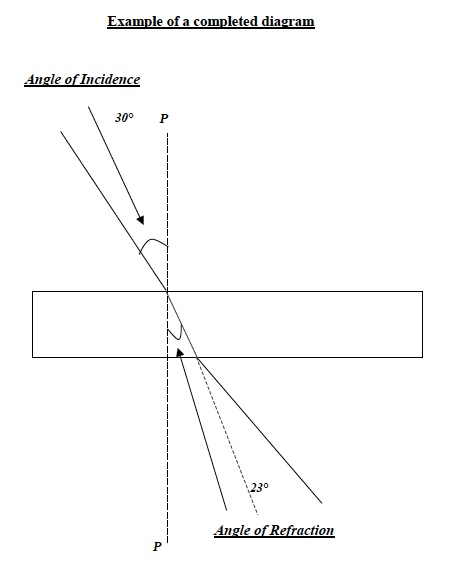1. Calculate: Use the following formula to calculate the Index of Refraction (nr) of the acrylic semicircle:1. Record: Record your calculations in Table A below.

Trial 2: Refraction through water

1. Use the same procedure from before to approximate the index of refraction of water.

A. In order to determine the index of refraction of the water, fill the refraction tank halfway with water.

B. Although light is entering glass and the water, most of the refraction will be due to the water inside the refraction tank, rather than the glass.

2. Create the diagram of the refraction of light through water on a separate sheet of paper.

Note: A sample diagram is provided below to help you with the procedure.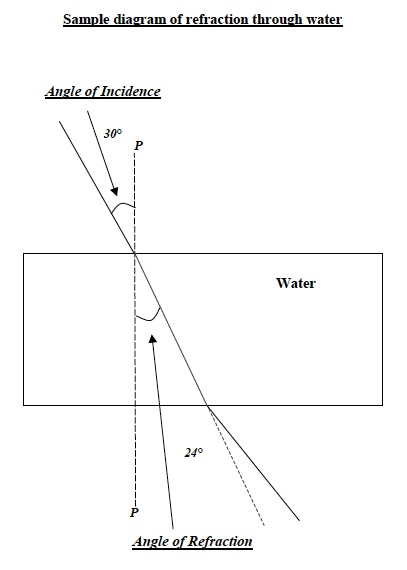1. Calculate: Use the following formula to calculate the Index of Refraction (nr) of the water:1. Record: Record your calculations in Table A.

Trial 3: Refraction through oil

1. Use the same procedure from before to approximate the index of refraction of oil.

A. In order to determine the index of refraction of the oil, fill the refraction tank halfway with oil.

B. Although light is entering glass and the oil, most of the refraction will be due to the oil inside the refraction tank, rather than the glass.

2. Create the diagram of the refraction of light through oil on a separate sheet of paper.

Note: A sample diagram is provided below to help you with the procedure.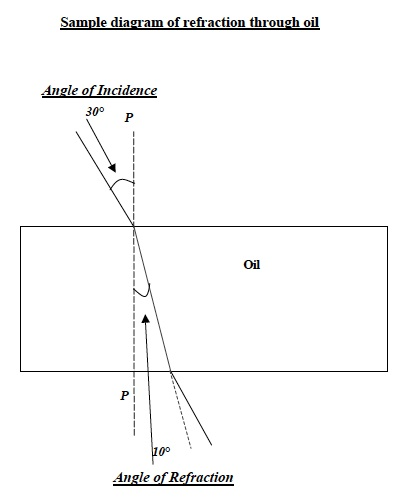1. Calculate: Use the following formula to calculate the Index of Refraction (nr) of the oil:1.  Record: Record your calculations in Table A below.Note: Questions 5-7 are included as part of the PostLab Analysis. They are also included here for reference.

1. Which medium produced the greatest refraction of light? Use data to support your answer.
1. How does the index of refraction affect the amount that light waves are bent or refracted?
1. When you observed the pins through the semicircle, the water, and the oil did they appear different than when viewed without the semicircle, water, and oil? Why?
1. Is the refraction of light ever visible? Can you observe refraction more readily in some circumstances than others? Think about these questions and then compare how objects look through the rectangular refraction tank and acrylic semicircle to how objects appear through lenses.
1. Compare a flat piece of glass with a curved piece:

A. Combine with another pair and obtain the following materials: 1 glass slide, 1 concave lens, and 1 convex lens.

B. Look at the following figure without and then through a glass slide. What do you observe?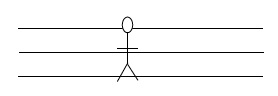C. Look at the figure in the previous question using the semicircle, concave, and convex lens.

D. How does the figure appear when viewed with and without the lenses and semicircle?

1. Test for signs of refraction using a second method.

A. Place the glass slide on edge lengthwise along an invisible line connecting the letters A and B on the diagram.

B. Look at the lines on the left side of points A and B.

C. Look through the glass slide to the right side of points A and B.

D. Observe the lenses, glass slide, and semicircle. Feel each piece.

E. Repeat steps a through c with the concave and convex lenses.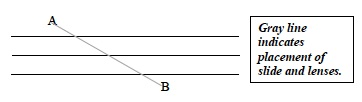F. What did you observe?

### CLEAN UP

Be sure to clean up your lab bench after you have finished your experiments.